Download Presentation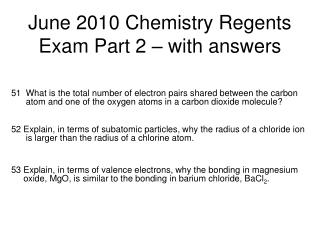June 2010 Chemistry Regents Exam Part 2 – with answers

# June 2010 Chemistry Regents Exam Part 2 – with answers - PowerPoint PPT Presentation

June 2010 Chemistry Regents Exam Part 2 – with answers. 51 What is the total number of electron pairs shared between the carbon atom and one of the oxygen atoms in a carbon dioxide molecule?I am the owner, or an agent authorized to act on behalf of the owner, of the copyrighted work described.
Download Presentation## June 2010 Chemistry Regents Exam Part 2 – with answers

An Image/Link below is provided (as is) to download presentation

Download Policy: Content on the Website is provided to you AS IS for your information and personal use and may not be sold / licensed / shared on other websites without getting consent from its author.While downloading, if for some reason you are not able to download a presentation, the publisher may have deleted the file from their server.

- - - - - - - - - - - - - - - - - - - - - - - - - - E N D - - - - - - - - - - - - - - - - - - - - - - - - - -
Presentation Transcript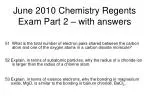### June 2010 Chemistry Regents Exam Part 2 – with answers

51 What is the total number of electron pairs shared between the carbon atom and one of the oxygen atoms in a carbon dioxide molecule?

52 Explain, in terms of subatomic particles, why the radius of a chloride ion is larger than the radius of a chlorine atom.

53 Explain, in terms of valence electrons, why the bonding in magnesium oxide, MgO, is similar to the bonding in barium chloride, BaCl2.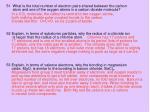51 What is the total number of electron pairs shared between the carbon atom and one of the oxygen atoms in a carbon dioxide molecule?

In a CO2 molecule, the carbon is central to two oxygen atoms, both making double polar covalent bonds to the carbon. It looks like this: O=C=O so it’s 2 pairs of bonds.

52 Explain, in terms of subatomic particles, why the radius of a chloride ion is larger than the radius of a chlorine atom. Chlorine has 17 protons and 17 electrons and is one of the smallest atoms in its period (lots of protons for 3 orbitals, only the noble gas Ar is smaller). The chloride anion still has 17 protons, but has 18 electrons, a full outer most orbital. The anion is slightly stretched out, the extra negative charge making is push as large as it can be.

53 Explain, in terms of valence electrons, why the bonding in magnesium oxide, MgO, is similar to the bonding in barium chloride, BaCl2. Magnesium from group 2 must transfer away 2 electrons and oxygen needs to pick up two electrons to get a full orbital. Ionic compounds must transfer a perfect number of electrons, no left over electrons, no IOU electrons. Barium is also in group two, and must also transfer away 2 electrons. When bonding to chlorine anions which only pick up one electron each, it takes 2 chloride anions to pick up these two electrons. Both Mg and Ba cations are +2 cations in neutral ionic compounds.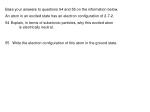• An atom in an excited state has an electron configuration of 2-7-2.
• Explain, in terms of subatomic particles, why this excited atom is electrically neutral.
• 55 Write the electron configuration of this atom in the ground state.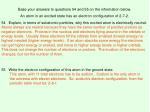An atom in an excited state has an electron configuration of 2-7-2.

54 Explain, in terms of subatomic particles, why this excited atom is electrically neutral. Atoms always are neutral because they have the same number of positive protons as negative electrons. Protons in the nucleus and electrons flying around in the orbitals or energy levels. Usually these electrons fill the orbitals from smallest (lowest energy) to highest needed (higher energy levels). If an atom absorbs some energy the electrons can get higher energy or excited, and move to higher energy levels or higher orbitals. This does not change the number of electrons or protons. The location of the electrons changes, but not the number of them.

55 Write the electron configuration of this atom in the ground state. This atom, with 11 total electrons has to be sodium. Sodium is the only atom in the universe with eleven electrons. So, sodium’s electron configuration, from the periodic table must be: 2-8-1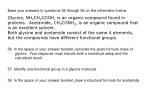Base your answers to questions 56 through 58 on the information below.

Glycine, NH2CH2COOH, is an organic compound found in proteins. Acetamide, CH3CONH2, is an organic compound that is an excellent solvent. Both glycine and acetamide consist of the same 4 elements, but the compounds have different functional groups.

56 In the space in your answer booklet, calculate the gram-formula mass of glycine. Your response must include both a numerical setup and the calculated result.

57 Identify one functional group in a glycine molecule.

58 In the space in your answer booklet, draw a structural formula for acetamide.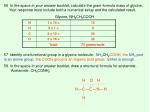56 In the space in your answer booklet, calculate the gram-formula mass of glycine. Your response must include both a numerical setup and the calculated result.

Glycine, NH2CH2COOH

57 Identify one functional group in a glycine molecule. NH2CH2COOH, the NH2 part is an amine group, the COOH group is an organic acid group. Either is enough.

58 In the space in your answer booklet, draw a structural formula for acetamide. Acetamide, CH3CONH2

H

O

H

H―C―C―N―H

H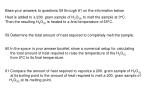Base your answers to questions 59 through 61 on the information below.

Heat is added to a 200. gram sample of H2O(S) to melt the sample at 0°C. Then the resulting H2O(L) is heated to a final temperature of 65°C.

59 Determine the total amount of heat required to completely melt the sample.

60 In the space in your answer booklet, show a numerical setup for calculating the total amount of heat required to raise the temperature of the H2O(L) from 0°C to its final temperature.

61 Compare the amount of heat required to vaporize a 200. gram sample of H2O(L) at its boiling point to the amount of heat required to melt a 200. gram sample of H2O(S) at its melting point.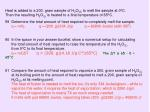Heat is added to a 200. gram sample of H2O(S) to melt the sample at 0°C. Then the resulting H2O(L) is heated to a final temperature of 65°C.

59 Determine the total amount of heat required to completely melt the sample. q = mHF q = (200. g)(334 J/g) q = 66800 Joules (with 3SF)

60 In the space in your answer booklet, show a numerical setup for calculating the total amount of heat required to raise the temperature of the H2O(L) from 0°C to its final temperature. q = mCΔT q = (200. g)(4.18 J/g·C°)(65°C) The ΔT is 65 – 0 = 65°C

61 Compare the amount of heat required to vaporize a 200. gram sample of H2O(L) at its boiling point to the amount of heat required to melt a 200. gram sample of H2O(S) at its melting point. The heat of fusion is needed to melt the ice, it’s only 334 Joules/gram – while to vaporize this water, it requires the heat of vaporization, which is 2260 J/gm. The heat of vaporization is nearly 7X as much energy needed. It melts with just 66800 Joules, vaporizing takes (200. g)(2260 J/g) = 452000 J!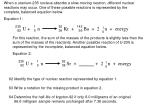When a uranium-235 nucleus absorbs a slow-moving neutron, different nuclear reactions may occur. One of these possible reactions is represented by the complete, balanced equation below.

Equation 1:

23592

10

9236

14256

U +

n

Kr +

Ba + 2

10

n + energy

For this reaction, the sum of the masses of the products is slightly less than the sum of the masses of the reactants. Another possible reaction of U-235 is represented by the incomplete, balanced equation below.

Equation 2:

23592

10

9238

U +

n

Sr +

+ 2

10

n + energy

62 Identify the type of nuclear reaction represented by equation 1.

63 Write a notation for the missing product in equation 2.

64 Determine the half-life of krypton-92 if only 6.0 milligrams of an original 96.0-milligram sample remains unchanged after 7.36 seconds.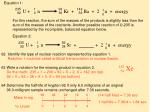Equation 1:

23592

10

9236

14256

U +

n

Kr +

Ba + 2

10

n + energy

For this reaction, the sum of the masses of the products is slightly less than the sum of the masses of the reactants. Another possible reaction of U-235 is represented by the incomplete, balanced equation below.

Equation 2:

23592

10

9238

U +

n

Sr +

+ 2

10

n + energy

62 Identify the type of nuclear reaction represented by equation 1. Reaction 1 could be called artificial transmutation or nuclear fission.

63 Write a notation for the missing product in equation 2. Do the math: 235 + 1 = 236 = 92 + X + 2, X = 142 (on top) 92 + 0 = 92 = 38 + X + 0, X = 54 (on bottom)

64 Determine the half-life of krypton-92 if only 6.0 milligrams of an original 96.0-milligram sample remains unchanged after 7.36 seconds.

14254

Xe

96 48 24 12 6

If 4 half lives = 7.36 sec

1 half life = 7.36/4 = 1.84 sec

Mass in mg

½ Lives

0 1 2 3 4Base your answers to questions 65 through 68 on the information below.

In a laboratory, a student makes a solution by completely dissolving 80.0 grams of KNO3(S) in 100.0 grams of hot water. The resulting solution has a temperature of 60.°C.

The room temperature in the laboratory is 22°C.

65 Classify, in terms of saturation, the type of solution made by the student.

66 Compare the boiling point of the solution at standard pressure to the boiling point of water at standard pressure.

67 Describe the direction of heat flow between the solution made by the student and the air in the laboratory.

68 Describe a laboratory procedure that can be used to recover the solid solute from the aqueous solution.In a laboratory, a student makes a solution by completely dissolving 80.0 grams of KNO3(S) in 100.0 grams of hot water. The resulting solution has a temperature of 60.°C. The room temperature in the laboratory is 22°C.

65 Classify, in terms of saturation, the type of solution made by the student. According to table G, at 60°C, 100 mL water can hold approx. 105 g KNO3. This solution is therefore unsaturated.

66 Compare the boiling point of the solution at standard pressure to the boiling point of water at standard pressure. Pure water would have a BP of 100°C at standard pressure. The more solute dissolved into water, the higher the BP becomes. This change in this colligative property is due to additional internal attraction. The water molecules are attracted to themselves and the ions in solution too.

67 Describe the direction of heat flow between the solution made by the student and the air in the laboratory. Heat flows from hot to cold, so heat flow will go from the hotter solution into the air of the lab.

68 Describe a laboratory procedure that can be used to recover the solid solute from the aqueous solution. Mixtures can be separated by physical means, you could boil away the water, leaving behind the solute, or you could distill it, saving solute as well as the water.Base your answers to questions 69 through 71 on the information below.

At room temperature, a reaction occurs when KIO3(AQ) is mixed with NaHSO3(AQ) thatcontains a small amount of starch. The colorless reaction mixture turns dark blue after aperiod of time that depends on the concentration of the reactants.

In a laboratory, 12 drops of a 0.02 M NaHSO3(AQ) solution containing starch wereplaced in each of six test tubes. A different number of drops of 0.02 M KIO3(AQ) andenough water to maintain a constant volume were added to each test tube and the time for the dark-blue color to appear was measured. The data were recorded in the table below.

69 Mark an appropriate scale on the axis labeled “Time (s).” Plot the data from the data table. Circle and connect the points.

70 State how increasing the number of drops of 0.02 M KIO3(AQ) used in the reaction affects the rate of reaction.

71 Identify one factor, other than the concentration of the reactants, that would affect the rate of this reaction.Reaction Time as a function of # drops of KIO3 in lab

220

200

180

160

140

120

100

80

60

40

20

0

Time in seconds

The dots should be connected with a smooth curved line, but I can’t draw that on a computer.

0 2 4 6 8 10 12

# of drops70 State how increasing the number of drops of 0.02 M KIO3(AQ) used in the reaction affects the rate of reaction. The more drops the less time this reaction will take because of the increased number of collisions (but that DOES NOT answer this question. Increased drops will increase the rate of reaction (=less time)

71 Identify one factor, other than the concentration of the reactants, that would affect the rate of this reaction. The four factors that increase the rates of reactions are: Increase temperature (more kinetic energy yields more collisions,

Increase concentration of reactants (also, more collisions would occur)

Increase surface area (more surface, more collisions)

And, a catalyst, which does not increase collisions of reactants, but offers a lower energy, alternate pathway for the reaction or happen (or it lowers activation energy required to start reaction.Base your answers to questions 72 through 75 on the information below.

The Solvay process is a multistep industrial process used to produce washing soda,Na2CO3(S). In the last step of the Solvay process, NaHCO3(S) is heated to 300°C, producing washing soda, water, and carbon dioxide. This reaction is represented by the balanced equation below.

2NaHCO3(S) + heat → Na2CO3(S) + H2O(G) + CO2(G)

72 Write the IUPAC name for washing soda.

73 Identify the type of chemical reaction represented by the equation.

74 State evidence that indicates the entropy of the products is greater than the entropy of the reactant.

75 Determine the total mass of washing soda produced if 3360. kilograms of NaHCO3 reacts completely to produce 360. kilograms of H2O and 880. kilograms of CO2.Base your answers to questions 72 through 75 on the information below.

The Solvay process is a multistep industrial process used to produce washing soda,Na2CO3(S). In the last step of the Solvay process, NaHCO3(S) is heated to 300°C, producing washing soda, water, and carbon dioxide. This reaction is represented by the balanced equation below.

2NaHCO3(S) + heat → Na2CO3(S) + H2O(G) + CO2(G)

72 Write the IUPAC name for washing soda. Na2CO3(S) is sodium carbonate

73 Identify the type of chemical reaction represented by the equation. This is a decomposition reaction

74 State evidence that indicates the entropy of the products is greater than the entropy of the reactant. the products contain smaller particles, and more importantly gases, which have much more entropy than solids

75 Determine the total mass of washing soda produced if 3360. kilograms of NaHCO3 reacts completely to produce 360. kilograms of H2O and 880. kilograms of CO2. This is just a conservation of matter problem, do the math: 3360. kg = X kg washing soda + 360. kg water + 880. kg CO2.

3360 = X + 360 + 880 X = 2120 X = 2120 kg washing sodaBase your answers to questions 76 through 79 on the information below.

In liquid water, an equilibrium exists between H2O(L) molecules, H+(AQ) ions, and OH−(AQ) ions. A person experiencing acid indigestion after drinking tomato juice can ingest milk of magnesia to reduce the acidity of the stomach contents. Tomato juice has a pH value of 4. Milk of magnesia, a mixture of magnesium hydroxide and water, has a pH value of 10.

76 Complete the equation in your answer booklet for the equilibrium that exists in liquid water.

77 Compare the hydrogen ion concentration in tomato juice to the hydrogen ion concentration in milk of magnesia.

78 Identify the negative ion found in milk of magnesia.

79 What is the color of thymol blue indicator when placed in a sample of milk of magnesia?Base your answers to questions 76 through 79 on the information below.

In liquid water, an equilibrium exists between H2O(L) molecules, H+(AQ) ions, and OH−(AQ) ions. A person experiencing acid indigestion after drinking tomato juice can ingest milk of magnesia to reduce the acidity of the stomach contents. Tomato juice has a pH value of 4. Milk of magnesia, a mixture of magnesium hydroxide and water, has a pH value of 10.

76 Complete the equation in your answer booklet for the equilibrium that exists in liquid water. H2O(L) H+1(AQ) + OH−1 (AQ)

77 Compare the hydrogen ion concentration in tomato juice to the hydrogen ion concentration in milk of magnesia. Tomato juice is an acid, it has a lot more hydrogen ions in it that the base called milk of magnesia. A difference in pH value between 4 to 10 is a 10x10x10x10x10x10= a 1 million times more H+ ions

78 Identify the negative ion found in milk of magnesia. The base anion is OH-1 or the hydroxide anion

79 What is the color of thymol blue indicator when placed in a sample of milk of magnesia? milk of magnesia with a pH = 10 would make thymol blue: BLUEBase your answers to questions 80 through 83 on the information below.

Two sources of copper are cuprite, which has the IUPAC name copper (I) oxide, and malachite, which has the formula Cu2CO3(OH)2. Copper is used in home wiring and electric motors because it has good electrical conductivity. Other uses of copper not related to its electrical conductivity include coins, plumbing, roofing, and cooking pans.

Aluminum is also used for cooking pans. At room temperature, the electrical conductivity of a copper wire is 1.6 times greater than an aluminum wire with the same length and cross-sectional area. At room temperature, the heat conductivity of copper is 1.8 times greater than the heat conductivity of aluminum.

At STP, the density of copper is 3.3 times greater than the density of aluminum.

80 Write the chemical formula of cuprite.

81 Determine the oxidation number of oxygen in the carbonate ion found in malachite.

82 Identify one physical property of copper that makes it a good choice for uses that are not related to electrical conductivity.

83 Identify one physical property of aluminum that could make it a better choice than copper for a cooking pan.81 Determine the oxidation number of oxygen in the carbonate ion found in malachite.The only oxidation number for oxygen is -2

82 Identify one physical property of copper that makes it a good choice for uses that are not related to electrical conductivity. Copper is very malleable, good conductor of heat, high melting point (one of the worst questions ever on a regents exam, according to me)

83 Identify one physical property of aluminum that could make it a better choice than copper for a cooking pan. An aluminum pan has less mass than a copper pan of the same size because aluminum is less dense. Aluminum is less dense than copper. A copper pan would weigh more. (that is until this particular question)

Sometimes the regents tries too hard to “connect” chemistry to the real world. Since I am “so strong”, a heavier copper pan would not be worse, nor would an aluminum one be too light. All metals are (relatively) good conductors of heat. These were awful!) Besides, good cooks are all quirky, they can’t be all the same!